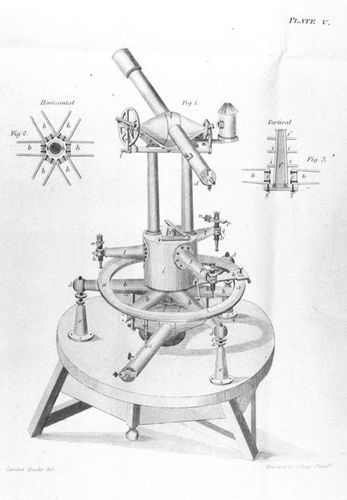# 12.4: Azimuth and Elevation

•• Contributed by CK12
• CK12Figure $$\PageIndex{1}$$: An old surveyor's telescope (theodolite).

The surveyor's telescope is designed to measure two such angles. The angle $$\phi$$ is measured counterclockwise in a horizontal plane, but surveyors (and soldiers) work with azimuth, a similar angle measured clockwise from north. Thus the directions (north, east, south, west) have azimuth (0°, 90°, 180°, 270°). A rotating table allows the telescope to be pointed in any azimuth.

The angle $$\lambda$$ is called elevation and is the angle by which the telescope is lifted above the horizontal (if it looks down, $$\lambda$$ is negative). The two angles together can in principle specify any direction: $$\phi$$ ranges from 0 to 360, and $$\lambda$$ from –90 (straight down or “nadir") to +90 (straight up or “zenith").

Again, one needs to decide from what direction is the azimuth measured--that is, where is azimuth zero? The rotation of the heavens (and the fact most humanity lives north of the equator) suggests (for surveyor-type measurements) the northward direction, and this is indeed the usual zero point. The azimuth angle (viewed from the north) is measured counterclockwise.